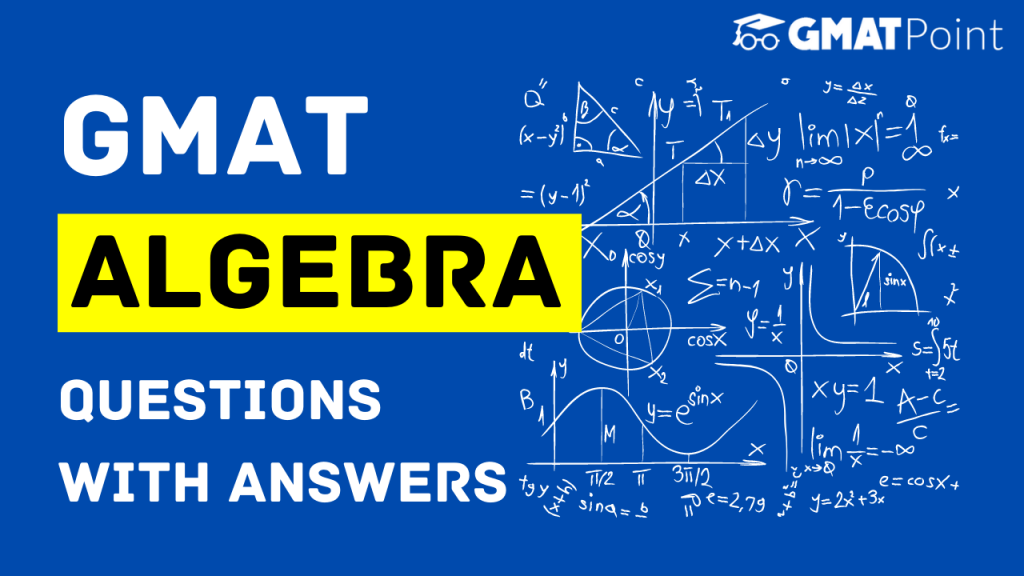# GMAT Algebra Questions With Answers# GMAT Algebra Questions With Answers

Algebra questions constitute a major portion of the Quant section of the GMAT. These questions can come under both Problem Solving and Data Sufficiency types. Algebra has a number of topics in it. In this article, we will be looking into –

1. GMAT Algebra Topics
2. GMAT Algebra Questions with Answers

## GMAT Algebra Topics

In the GMAT, Algebra constitutes a number of topics. They are as follows (this list is in accordance with the GMAT Official Guide):

1. Simplifying Algebraic Expressions
2. Equations
3. Linear Equations
5. Exponents
6. Inequalities
7. Absolute value or modulus
8. Functions

## Examples – GMAT Algebra Questions

### Question 1:

Is xy < 6 ?

Statement 1: x< 2, y<3

Statement 2: x and y are non-negative

### Approach:

Let us consider Statement 1. If x < 2 and y < 3, then xy < 6. But this is only possible if we do not consider the sign.

Imagine that one of them turns out to be negative, then irrespective of the other value, xy < 6.

However, if both turn out to be negative, then xy can take any value as large as possible.

Hence, Statement 1 is not sufficient.

Statement 2 is again not sufficient since x and y can take any value.

When we combine these statements, we know that x and y are either positive or zero. If any one or both are zero, xy < 6

When none of them is zero, they are still less than 6.

Hence, option C stating that both together are sufficient, however, none of them individually are sufficient is right.

### Question 2:

For how many integer values of x does the following inequality hold true?

(x-2)(x-4)(x-6)(x-8) < 0

### Approach:

In these questions, we take the boundary points. In this case, they are

2, 4, 6, 8

Let’s say the region where x > 8, the expression is positive.

Now, we can change signs at boundaries if the power of the term (x – a) where a is the point of the boundary is odd, else we retain the sign.

Hence, from 6 to 8, it is negative.

Again from 4 to 6, it is positive.

From 2 to 4, it is negative.

For x < 2, it is positive.

Hence, for a total of 2 values – 3 and 7 – the inequality holds true.

### Question 3:

A function f(x) is defined for all natural numbers such that

f(x) = x + 1  [x is even]

f(x) = x – 1 [x is odd]

The range of values of f(x) does not include how many natural numbers?

### Approach:

f(1) = 0

f(2) = 3

f(3) = 2

f(4) = 5

f(5) = 4

…..

…..

This way, all the natural numbers are covered except for 1.

Hence, only 1 is not present in the range of values that represent the range of f(x).

### Note:

Though these examples provide a good sense of what type of GMAT Algebra questions you can expect, in no way do they represent the exhaustive list of concepts required for the Quantitative section of GMAT.

### Tips to keep in mind:

1. Try to take some time out from the Problem-Solving Algebra questions so that you can use that time to solve the tricky Data Sufficiency Algebra questions.
2. Do not get stuck in a question for long. If you find yourself trapped in a question for long, take a guess and move on.